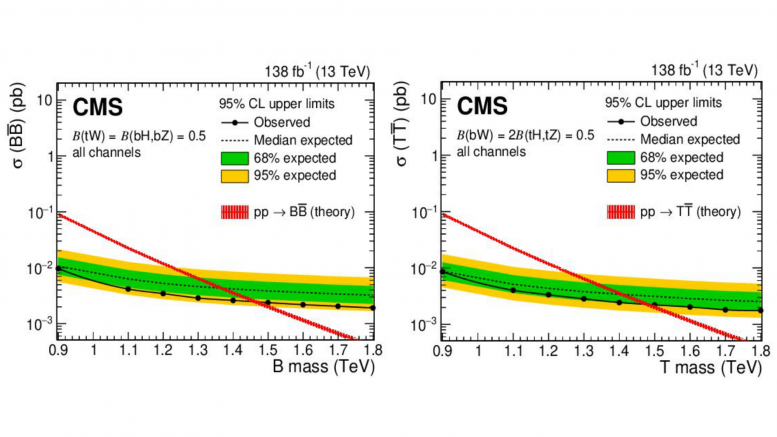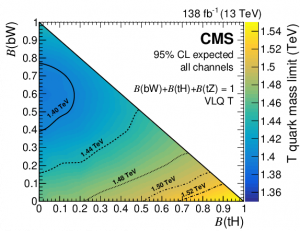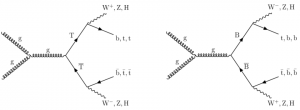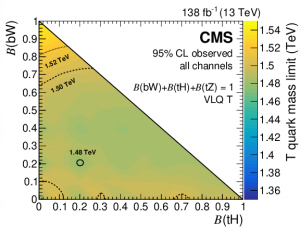# Limits Set: Vector-Like Quark Pair ProductionThe upper limits with $$95\%$$ confidence level (CL) for observed (solid lines) and median expected (dashed lines) for vector-like quark production. Singlet production cross sections for $$\text{B}\overline{\mbox{B}}$$ (left) and $$\text{T}\overline{\mbox{T}}$$ (right) are shown with a combined fit to all channel hypotheses. Theoretical cross sections depicted by the red line, with the fainter, surrounding band representing uncertainties in the calculation from particle distribution function (PDF) and energy scale calculations | Source: CMS

In mid-September of 2022, physicists from the CMS Collaboration at CERN reported new lower limits on vector-like top $$(\text{T})$$ and bottom $$(\text{B})$$ quarks. The analysis offers the strongest identified limits on the masses for both theoretical particles in a pair-production model $$(\text{T}\overline{\mbox{T}}, \text{B}\overline{\mbox{B}} )$$, with $$\text{T}$$ decaying to all third-generation quark decays $$(\text{t,b})$$ for the former (dropping the limit to $$1.48 \text{ TeV}$$ depending on analysis sensitivity to top quarks) and $$\text{B} \to \text{tW}$$ decays for the latter.

Beyond the Standard Model

Since the 2011-2012 ATLAS and CMS Collaborations’ discovery of the Higgs boson at a mass of $$125.35 \pm 0.15 \text{ GeV}$$ at CERN, physicists deemed the Standard Model (SM) to be as complete as possible within its major theoretical predictions. The confirmation of the Higgs field’s existence explained the electroweak spontaneous symmetry breaking found in the Standard Model’s $$SU(3)_C \times SU(2)_L \times U(1)_Y$$ gauge symmetry, filling the mathematical holes in certain SM-based predictions of massless particles. High-precision measurements and analyses of elementary particle physics phenomena continue to align with the values predicted by the SM, yielding a rigorous framework for particle physics research.The expected (theoretical) $$95\%$$ CL lower limits for mass in $$\text{T}$$ quark pair production as a function of its branching ratio to $$\text{H}$$ and $$\text{W}$$ bosons, formatted as a mass contour | Source: CMS

Yet, occasional experimental signs of non-SM behavior, especially in neutrino oscillation experiments such as MicroBooNE, alongside various unexplained phenomena, including gravitational interactions, motivate research for “beyond the Standard Model” (BSM) theories. One prevalent approach among proposed BSM theories is to introduce further, heavier particles with decoupling, essentially smoothing out certain mass terms of the SM Lagrangian (the mathematical energy relations describing behaviors of SM fields). Certain decoupling theories propose the addition of a sequential family of fermions (increasing generations from 3 to 4), relying on the standard Higgs Yukawa couplings for determining mass. However, the reliance on Higgs Yukawa couplings implies that the masses can not be taken high enough while both avoiding any constraints of contemporary collider technology and keeping calculations in line with the SM’s numerous measurements.

Thus, introducing pairs of heavier, chiral-symmetric fermions into the model allows the mass term to not depend on Higgs Yukawa couplings, removing constraints on their mass.

VLQ Chiral Symmetry

The quarks of the Standard Model do not have a parity (also dubbed chirality or “handedness”) symmetry under the SM gauge group. This means that left-handed and right-handed quarks behave differently under weak interactions, where only the former (and antiparticles of these left-handed quarks, as the transformation affects all conservation numbers including parity) sees effects from the weak force’s SM gauge bosons: $$\text{Z, W}^{\pm}$$.  On the other hand, vector-like quarks (VLQ’s), arising from theories such as supersymmetry (SUSY), little Higgs, and composite Higgs, would have their left and right-handed components transform under identical representations of $$SU(2)$$, the weak force symmetry group.

Decay Mechanisms

The minimal theoretical models (offering the least mathematical deviation from the current SM fermionic theory) for VLQ production yield heavier, fourth-generation top $$(\text{T})$$ and bottom $$(\text{B})$$ quarks, in analog to the SM top and bottom. These $$\text{T}$$ and $$\text{B}$$ are assumed to have third-generation mixing with the SM quarks, aligning with the predictions of large top $$(\text{t})$$ quark couplings.Tree-level Feynman diagrams depicting QCD pair production of $$\text{T}$$ (left) and $$\text{B}$$ (right) pair production decaying to third generation SM quarks and heavy SM bosons | Source: CMS

Given the parameters of the Large Hadron Collider (LHC) at CERN, VLQs pair produce through a gluonic strong (QCD) interaction or in single particle production through electroweak interactions. The $$\text{T}$$ has an electric charge of $$2e/3$$ and the $$\text{B}$$ has a charge of $$-e/3$$. Assuming the minimal models, the VLQs can arise as electroweak singlets $$($$either a $$\text{T}$$ or a $$\text{B})$$, in doublet form as $$\text{T, B}$$, or in other doublet and triplet combinations with other exotic VLQs with differing charges. As expected, the decay branching ratios are unique for distinct singlet (or doublet/ triplet) scenarios. Predicted branching ratios are as follows:

$$\begin{array}{ c | c c } & \text{Singlets} & \text{Doublets} \\ \hline \text{T} \to \text{bW} & 50\% & \text{n/a} \\ \hline \text{T} \to \text{tH} & 25\% & 50\% \\ \hline \text{T} \to \text{tZ} & 25\% & 50\% \\ \hline \text{B} \to \text{tW} & 50\% & 50\% \\ \hline \text{B} \to \text{bH} & 25\% & \text{n/a} \\ \hline \text{B} \to \text{bZ} & 25\% & 50\% \\ \end{array}$$

The singlet and doublet branching fractions stood as a benchmark reference for the search, selecting three leptonic final states to eliminate the statistical background and set lower limits on the masses of the $$\text{T}$$ and $$\text{B}$$.

Final States and Results

The analysis was conducted using LHC data from proton-proton $$(\text{pp})$$ collisions at $$\sqrt{s}=13\text{ TeV}$$ at an integrated luminosity of $$138\text{ fb}^{-1}$$ between 2016 and 2018 at the CMS experiment. The collaboration used three leptonic final states consisting of charged electrons and muons (as taus pose decay width and detection difficulties): single-lepton, same-signed dilepton, and multilepton $$(n\geq 3)$$. Same-signed dilepton pairs $$(e^{\pm}e^{\pm},\mu^{\pm}\mu^{\pm})$$ occur less frequently than oppositely-signed dilepton production $$(e^{\pm}e^{\mp},\mu^{\pm}\mu^{\mp})$$, thus providing the analysis with a more distinct signal that can be easier to identify while sifting through background decays. Additionally, the physicists assumed only a single VLQ flavor, either $$\text{T}$$ or $$\text{B}$$, was present in a given decay, as the predicted production cross section is low enough to confidently exclude channels involving both VLQs. Thus, the mass lower limits and confidence level results were determined using independent analyses for each particle.The observed (experimental) $$95\%$$ CL lower limits for mass in $$\text{T}$$ quark pair production as a function of its branching ratio to $$\text{H}$$ and $$\text{W}$$ bosons, formatted as a mass contour | Source: CMS

Each channel involved various algorithmic analyses. The single-lepton channel used a common high energy physics (HEP) jet algorithm to identify the parent (decayed from) particles for jets with large radii, followed by VLQ candidate reconstruction using a multilayer perception network. The same-sign dilepton and multilepton channels exploited the rarity of same-signed leptonic pair production as well as the relatively large energy signatures from desired signal events to study potential non-SM behavior. The CMS collaboration reported production of the $$\text{T}$$ and $$\text{B}$$ to be excluded for masses up to $$1.54 \text{ TeV}$$ and $$1.56 \text{ TeV}$$ with $$95\%$$ confidence, respectively.

Further analyses, especially after luminosity and detection upgrades to the LHC at CERN, will seek to investigate the VLQ limits beyond current limits. Though VLQs pose only a small fraction of BSM physical theories, the publication of strong $$(\text{T}\overline{\mbox{T}}, \text{B}\overline{\mbox{B}} )$$ limits acts to widen the experimental cutoffs for other heavy-fermionic theories. As exotic and BSM experimental searches continue to introduce new results, theoretical physicists gain more expansive data to build frameworks and alternative models, searching for new discoveries to “break” the Standard Model.